Courses

# NCERT Exemplar: Oscillations- 1 Notes | EduRev

## JEE : NCERT Exemplar: Oscillations- 1 Notes | EduRev

The document NCERT Exemplar: Oscillations- 1 Notes | EduRev is a part of the JEE Course NEET Revision Notes.
All you need of JEE at this link: JEE

MULTIPLE CHOICE QUESTIONS I

Q.1. The displacement of a particle is represented by the equation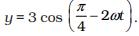The motion of the particle is
(a) Simple harmonic with period 2p/w.
(b) Simple harmonic with period π/ω.
(c) Periodic but not simple harmonic.
(d) Non-periodic.
Ans.
(b)
Solution.

• Acceleration is always directed towards the man position and so is always opposite to displacement, i.e. a ∝ -y or a = -ω2y
• The differential equation of S.H.M is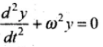and the solution of this differential equation is y = a cos(ωt + φ0)

Method 1: The displacement of the particle y = 3 cos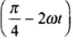Velocity of the particle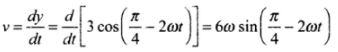Acceleration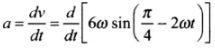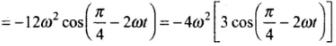Hence a = -4ω2y = -(2ω)2y
It means acceleration, a ∝ -y, the motion is SHM.
Hence angular frequency of S.H.M, ω' = 2ω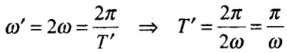It means the motion is SHM with period π/ω.
Method 2: Given the equation of displacement of the particle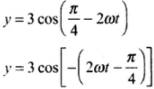We know cos(-θ) = cosθ
Hence y = 3cos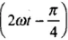...(i)
Comparing with y = a cos(ωt + ϕ0)
Hence (i) represents simple harmonic motion with angular frequency 2ω.
Hence its time period,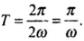Q.2. The displacement of a particle is represented by the equation y = sin3ωt. The motion is
(a) Non-periodic.
(b) Periodic but not simple harmonic.
(c) Simple harmonic with period 2π/ω.
(d) Simple harmonic with period π/ω.
Ans.
(b)
Solution.
There are certain motions that are repeated at equal intervals of time. Let the the interval of time in which motion is repeated. Then x(t) =x(t + T), where T is the minimum change in time. The function that repeats itself is known as a periodic function.
Given the equation of displacement of the particle, y = sin3ωt
We know sin 3θ = 3 sin θ - 4 sin3θ
Hence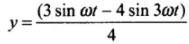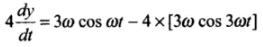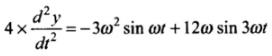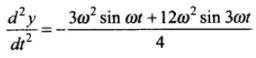⇒ d2y/dt2 is not proportional to y.
Hence, motion is not SHM.
As the expression is involving sine function, hence it will be periodic.
Also sin3ωt = (sin ωt)3
= [sin(ωt + 2π)]3 = [sinω(t + 2π/ω)]3
Hence, y = sin3ωt represents a periodic motion with period 2π/ω.

Q.3. The relation between acceleration and displacement of four particles are given below:
(a) ax = + 2x.
(b) ax = + 2x2.
(c) a= – 2x2.
(d) ax = – 2x.
Which one of the particles is executing simple harmonic motion?
Ans.
(d)
Solution.
In case of simple harmonic motion, the acceleration is always directed towards the mean position and so is always opposite to displacement, i.e. a α = -x or a = -ω2x
In option (d) ax = -2x or a α -x, the acceleration of the particle is proportional to negative of displacement.
Hence it represents S.H.M.

Q.4. Motion of an oscillating liquid column in a U-tube is
(a) Periodic but not simple harmonic.
(b) Non-periodic.
(c) Simple harmonic and time period is independent of the density of the liquid.
(d) Simple harmonic and time-period is directly proportional to the density of the liquid.
Ans.
(c)
Solution.
If the liquid in U-tube is filled to a height h and cross¬section of the tube is uniform and the liquid is incompressible and non- viscous. Initially the level of liquid in the two limbs will be at the same height equal to h. If the liquid is-pressed by y in one limb, it will rise by y along the length of the tube in the other limb, so the restoring force will be developed by hydrostatic pressure difference
Restoring force F = Weight of liquid column of height 2y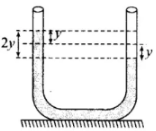⇒ F = -(A x 2y x ρ) x g = -2Aρgy
⇒ F ∝ -y
Motion is SHM with force constant
k = 2Aρg
⇒ Timeperiod T =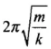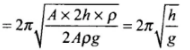which is independent of the density of the liquid.

Q.5. A particle is acted simultaneously by mutually perpendicular simple hormonic motions x = a cos ωt and y = a sin ωt . The trajectory of motion of the particle will be
(a) An ellipse.
(b) A parabola.
(c) A circle.
(d) A straight line.
Ans.
(c)
Solution.
If two S.H.M’s act in perpendicular directions, then their resultant motion is in the form of a straight line or a circle or a parabola etc. depending on the frequency ratio of the two S.H.Ms and initial phase difference. These figures are called Lissajous figures.
Let the equations of two mutually perpendicular S.H.M’s of same frequency be
x = a1 sin ωt and y = a2 sin( ωt + ϕ)
Then the general equation of Lissajou's figure can be obtained as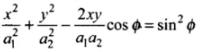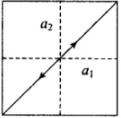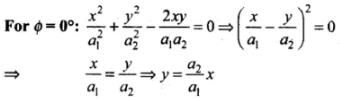This is a straight line which passes through origin and its slope is a2/a1.
Lissajou's Figures in other conditions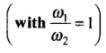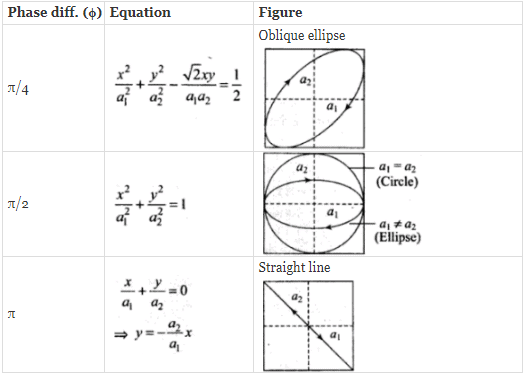We have to find resultant - displacement by adding x and y-components. According to variation of x and y trajectory will be predicted.
Given, x = a cos ωt = a sin (ωt (π/2) ...(i)
And y = a sin ωt ...(ii)
Here a1 = a2 = a and ϕ = π/2
The trajectory of motion of the particle will be a circle of radius a.

Q.6. The displacement of a particle varies with time according to the relation
y = a sin ωt + b cos ωt
(a) The motion is oscillatory but not S.H.M.
(b) The motion is S.H.M. with amplitude a + b.
(c) The motion is S.H.M. with amplitude a2 + b2.
(d) The motion is S.H.M. with amplitude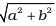.
Ans.
(d)
Solution.
y = a sin ωt + b cos ωt
The amplitude of motion is given by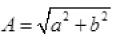Hence, verifies the option (d).

Q.7. Four pendulums A, B, C and D are suspended from the same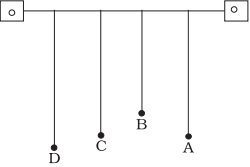elastic support as A and C are of the same length, while B is smaller than A and D is larger than A. If A is given a transverse displacement,
(a) D will vibrate with maximum amplitude.
(b) C will vibrate with maximum amplitude.
(c) B will vibrate with maximum amplitude.
(d) All the four will oscillate with equal amplitude.
Ans.
(b)
Solution.
Here A is given a transverse displacement. Through the elastic support the disturbance is transferred to all the pendulums.
A and C are having same length, hence they will be in resonance, because of their time period of oscillation. Since length of pendulums A and C is same  and T =2 π√L/g , hence their time period is same and they will have frequency of vibration. Due to it, a resonance will take place and the pendulum C will vibrate with maximum amplitude.

Q.8. Figure shows the circular motion of a particle. The radius of the circle, the period, sense of revolution and the initial position are indicated on the figure. The simple harmonic motion of the x-projection of the radius vector of the rotating particle P is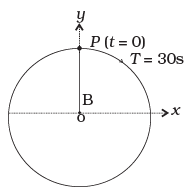(a)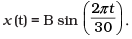(b)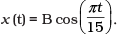(c)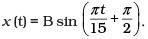(d)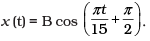Ans.
(a)
Solution.
Suppose a particle P is moving uniformly on a circle of radius A with angular speed. Q and R are the two feets of the perpendicular drawn from P on two diameters one along .Y-axis and the other along Y-axis.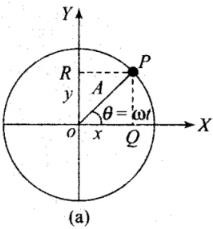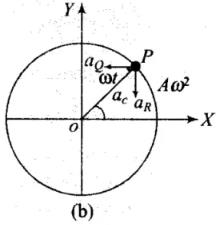Suppose the particle P is on the X-axis at t = 0. Radius OP makes an angle with the X-axis at time t, then x = A cosωt and y = A sinωt
Here, x and v are the displacements of Q and R from the origin at time t, which are the displacement equations of SHM. It implies that although P is under uniform circular motion, Q and R are performing SHM about O with the same angular speed as that of P.
Let angular velocity of the particle executing circular motion is ω and when it is at P makes an angle θ as shown is the diagram.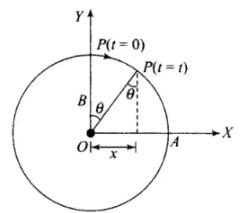As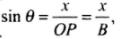Clearly, ω = ωt
x = B sin θ = B (sin ωt) = B sin(πt/15)
x = B sin (2π/30 (t))

Q.9. The equation of motion of a particle is x = a cos (α t)2. The motion is
(a) Periodic but not oscillatory.
(b) Periodic and oscillatory.
(c) Oscillatory but not periodic.
(d) Neither periodic nor oscillatory.
Ans.
(c)
Solution.
The equation of motion of a particle is
x = a cos(∝t)2
is a cosine function and x varies between -a and +a, the motion is oscillatory. Now checking for periodic motion, putting t+T in place of t. T is supposed as period of the function ω(t).
x(t + T) = a cos [α(t + T)]2
=  a cos[α2t2 + α2t2 + 2α2tT] ≠ x(t)
Hence, it is not periodic.

Q.10. A particle executing S.H.M. has a maximum speed of 30 cm/s and a maximum acceleration of 60 cm/s2. The period of oscillation is
(a) πs
(b) (π/2)s
(c) 2πs
(d) (π/t)s
Ans.
Consider a SHM equation of motion
y = a sin ωt
v = dy/dt = aω cos ωt ...(i)
a = dv/dt = -aw sin ωt
a = -aω2 sin ωt ...(ii)
vmax = 30 cm/s (given)
vmax from (i)
vmax = aω
∴ aω = 30 ...(iii)
amax = aω2 from(ii)
60 = aω2 ...(iv) (given)
60 = ω x 30
2π/T = 2
T = π. Verifies option (a).

Q.11. When a mass m is connected individually to two springs S1 and S2, the oscillation frequencies are ν1 and ν2. If the same mass is attached to the two springs as shown in Figure, the oscillation frequency would be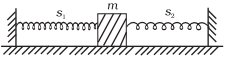(a) v1 + v2.
(b)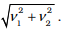(c)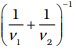(d)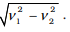Ans.
(b)
Solution.
When a mass (m) is connected to a spring on a horizontal frictionless surface then their frequencies are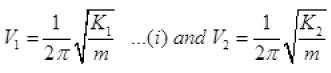Now, the spring are in parallel because one end of both spring are connected with rigid body and other end to body. So their equivalent spring constant becomes kP = K1 + K2
And frequency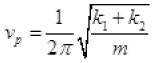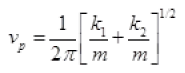Form (i)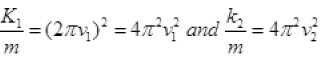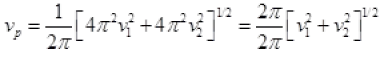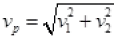Hence, verifies the option (b).

MULTIPLE CHOICE QUESTIONS II

Q.12. The rotation of earth about its axis is
(a) Periodic motion.
(b) Simple harmonic motion.
(c) Periodic but not simple harmonic motion.
(d) Non-periodic motion.
Ans.
(a, c)
Solution.
The motion of earth about its own axis is circular and complete its one complete revolution in regular interval of time. So it is periodic. But motion is not about a fixed point from which we can measure it’s displacement or about which it moves both side so it is not simple harmonic motion. So verifies the option (a) and (c).

Q.13. Motion of a ball bearing inside a smooth curved bowl, when released from a point slightly above the lower point is
(a) Simple harmonic motion.
(b) Non-periodic motion.
(c) Periodic motion.
(d) Periodic but not S.H.M.
Ans.
(a, c)
Solution.
For small angular displacement, the situation is shown in the figure. Only one restoring force creates motion in ball inside bowl.
Only one restoring force creates motion in ball inside bowl.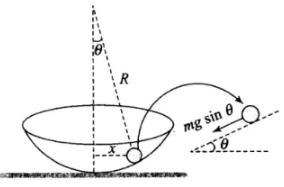F = -mg sinθ
As θ is small, sin θ = θ
So, ma = -mg(x/R)
or, a = -(g/R)x  ⇒ a ∝ -x
So, motion of the ball is S.H.M and periodic.

Q.14. Displacement vs. time curve for a particle executing S.H.M is shown in figure. Choose the correct statements.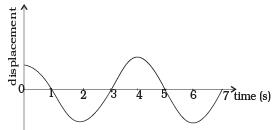(a) Phase of the oscillator is same at t = 0 s and t = 2 s
(b) Phase of the oscillator is same at t = 2 s and t = 6 s.
(c) Phase of the oscillator is same at t = 1 s and t = 7 s.
(d) Phase of the oscillator is same at t = 1 s and t = 5 s.
Ans.
(b, d)
Solution.
Two particles are said to be in same phase if the mode of vibration is same i.e., their distance will be nλ(/n = 1, 2, 3, ...)
(a) Distance between particles at t t = 0 and t = 2 is λ/2.
So, articles are not in same phase.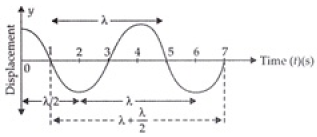(b) As from figure the particles at t = 2 sec and 6 sec are at distance λ, so are in same phase.
(c) Particles at t =1, t= 7 are the distance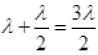so are not in phase.
(d) Particles at t = 1 sec and 5 sec are at distance = λ so are in same phase.

Q.15. Which of the following statements is/are true for a simple harmonic oscillator?
(a) Force acting is directly proportional to displacement from the mean position and opposite to it.
(b) Motion is periodic.
(c) Acceleration of the oscillator is constant.
(d) The velocity is periodic.
Ans.
(a, b, d)
Solution.
Consider a SHM x = a sin ωt. ...(i)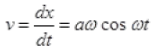...(ii)
Acceleration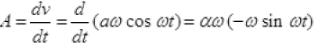∴ A = -αω2 sin ωt or A = ω2x
mA = -mω2x
F ∝ -(x)
Hence, force is directly proportional to displacement opposite the direction of displacement. We can prove
x(t) = x(t+T)
So motion is periodic and SHM.
From (ii) v is also periodic as we can prove
v(t) = v(t + T)
Hence, verifies the option

Q.16. The displacement time graph of a particle executing S.H.M is shown in figure. Which of the following statement is/are true?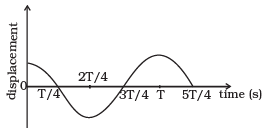(a) The force is zero at t = 3T/4.
(b) The acceleration is maximum at y = 4T/4.
(c) The velocity is maximum at t = T/4.
(d) The P.E. is equal to K.E. of oscillation at t = T/2.
Ans.
(a, b, c)
Solution.
(a) At t = 3T/4 Particle is at it’s mean position so force acting on it is zero, but it continue the motion due to inertia of mass, here a = 0, so F = 0.
(b) At t = 4T/4 = T, particle’s velocity changes increasing to decreases so maximum change in velocity at T. As acceleration = Change in velocity/Time, so acceleration is maximum here.
(c) At t = T/4 it is at its mean position where the velocity is maximum as no retarding force on it.
(d) t = T/2 = 2T/4, the particle has K.E = 0. So KE ≠ PE.
Hence, verifies the option (a,b,c)

Q.17. A body is performing S.H.M. Then its
(a) Average total energy per cycle is equal to its maximum kinetic energy.
(b) Average kinetic energy per cycle is equal to half of its maximum kinetic energy.
(c) Mean velocity over a complete cycle is equal to 2/π times of its maximum velocity.
(d) Root mean square velocity is 1/√2 times of its maximum velocity.
Ans.
(a, b, d)
Solution.
In case of S.H.M, average total energy per cycle
= Maximum kinetic energy (K0)
= Maximum potential energy (U0)
Average K.E per cycle =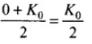Let us write the equation for the SHM x = a sin ωt.
Clearly, it is a periodic motion as it involves sine function.
Let us find velocity of the particle,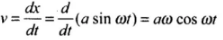Mean velocity over a complete cycle,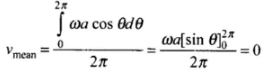So,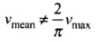Root mean square speed,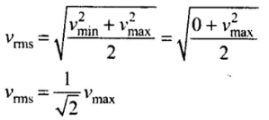Q.18. A particle is in linear simple harmonic motion between two points A and B, 10 cm apart. Take the direction from A to B as the + ve direction and choose the correct statements.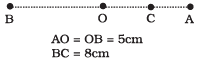(a) The sign of velocity, acceleration and force on the particle when it is 3 cm away from A going towards B are positive.
(b) The sign of velocity of the particle at C going towards O is negative.
(c) The sign of velocity, acceleration and force on the particle when it is 4 cm away from B going towards A are negative.
(d) The sign of acceleration and force on the particle when it is at point B is negative.
Ans.
(a, c, d)
Solution.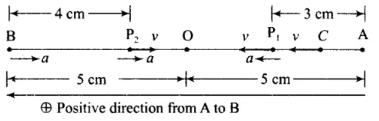In option (a): When the particle is 3 cm away from A going towards B. So, velocity is towards AB, i.e. positive. In SHM, acceleration is always towards mean position (O) it means both force and acceleration act towards O, have positive sign.
Hence option (a) is correct.
In option (b): When the particle is at C, velocity is towards B hence positive. Hence option (b) is not correct.
In option (c): When the particle is 4 cm away from B going towards A velocity is negative and acceleration is towards mean position (O), hence negative. Hence option (c) is correct.
In option (d): Acceleration is always towards mean position (O). When the particle is at B, acceleration and force are towards BA that is negative. Hence option (d) is correct.

Q.1. Displacement versus time curve for a particle executing S.H.M. is shown in Figure, Identify the points marked at which (i) velocity of the oscillator is zero, (ii) speed of the oscillator is maximum.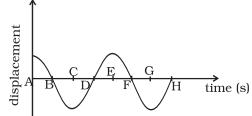Ans. In displacement-time graph of SHM, zero displacement values correspond to mean position; where velocity of the oscillator is maximum. Whereas the crest and troughs represent amplitude positions, where displacement is maximum and velocity of the oscillator is zero.
(i) The points A, C, E, G lie at extreme positions (maximum displacement, y = A). Hence the velocity of the oscillator is zero.
(ii) The points B, D, F, H lie at mean position (zero displacement, y = 0). We know the speed is maximum at mean position.

Q.2. Two identical springs of spring constant K are attached to a block of mass m and to fixed supports as shown in Fig. When the mass is displaced from equilibrium position by a distance x towards right, find the restoring force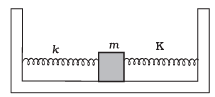Ans. When mass is displaced from equilibrium position by a distance x towards right, the right spring gets compressed by x developing a restoring force kx towards left on the block. The left spring is stretched by an amount x developing a restoring force kx left on the block.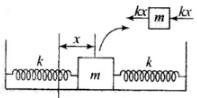developing a restoring force kx towards left on the block.
F1 = -kx (for left spring) and
F2 = -kx (for right spring)
Restoring force, F = F1 + F2 = -2kx
∴ F = 2kx towards left.

Q.3. What are the two basic characteristics of a simple harmonic motion?
Ans.
The two basic characteristics of a simple harmonic motion
(i) Acceleration is directly proportional to displacement.
(ii) The direction of acceleration is always towards the mean position, that is opposite to displacement.

Q.4. When will the motion of a simple pendulum be simple harmonic?
Ans.
Simple pendulum perform angular S.H.M. Consider the bob of simple pendulum is displaced through an angle θ shown. Q
The restoring torque about the fixed point O is
τ = mgl sinθ
If θ is small angle in radians, then sin θ =  0
⇒ mglθ
In vector form τ ∝ θ
Hence, motion of a simple pendulum is SHM for small angle of oscillations.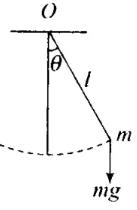Q.5. What is the ratio of maxmimum acceleration to the maximum velocity of a simple harmonic oscillator?
Ans.
Consider a SHM. x = A sin ωt
v = dx/dt = Aω cos ωt
For vmax cos ωt = -1
∴ vmax = Aω
a = dv/dt = -Aω2 sin ωt
For amax sing ωt = -1
amax = Aω2
∴ amax/vmax = Aω2/Aω = ω/1

Q.6. What is the ratio between the distance travelled by the oscillator in one time period and amplitude?
Ans.
In the diagram shown a particle is executing SHM between P and Q. The particle starts from mean position ‘O’ moves to amplitude position ‘P’, then particle turn back and moves from ‘P’ to 'Q'. Finally the particle turns back again and return to mean position ‘O’. In this way the particle completes one oscillation in one time period.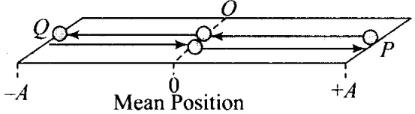Total distance travelled while it goes from O → P  →  O  →  Q  →  O
= OP + PO + OQ + QO = A + A + A + A = 4A
Amplitude = OP = A
Hence, ratio of distance and amplitude = 4A/A = 4

Q.7. In Figure, what will be the sign of the velocity of the point P′ , which is the projection of the velocity of the reference particle P. P is moving in a circle of radius R in anticlockwise direction.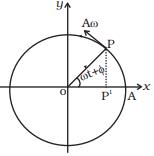Ans. As the particle on reference circle moves in anti-clockwise direction. The projection will move from P’ to O towards left, i.e. from right to left, hence sign is negative.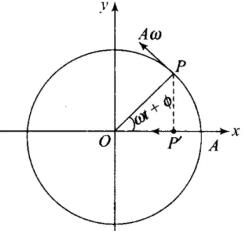Q.8. Show that for a particle executing S.H.M, velocity and displacement have a phase difference of π/2.
Ans.
Consider a SHM x = A sin ωt ...(i)
v = dx/dt = Aω cos ωt = Aω sin (90° + ωt)
(∵ sin (90° + θ) = cosθ)
∴ v = Aω sin (ωt + (π/2))
Phase of displacement from (i) is (ωt)
Phase of velocity from (ii) is (ωt + (π/2))
Hence, the phase difference = ωt +π/2 - ωt = π/2.

Q.9. Draw a graph to show the variation of P.E., K.E. and total energy of a simple harmonic oscillator with displacement.
Ans.
The potential energy (PE) of a simple harmonic oscillator is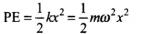...(i)
When, PE is plotted against displacement x, we will obtain a parabola.
When x = 0, PE = 0.
When x =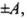PE = maximum
= 1/2 mω2A2
The kinetic energy (KE) of a simple harmonic oscillator KE = 1/2 mv2
But velocity of oscillator v =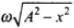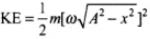or KE = 1/2mω2(A2-x2) ...(ii)
This is also parabola, if we plot KE against displacement x.
i.e., KE = 0 at x = ±A
and KE = 1/2 mω2A2at x = 0
Now, total energy of the simple harmonic oscillator = PE + KE [using Eqs. (i) and (ii)]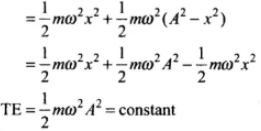Which is a constant and independent of x.
Plotting under the above guidelines KE, PE and TE versus displacement x-graph as follows: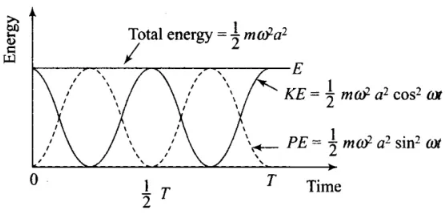Important point: From the graph we note that potential energy or kinetic energy completes two vibrations in a time during which S.H.M. completes one vibration. Thus the frequency of potential energy or kinetic energy is double than that of S.H.M.

Q.10. The length of a second’s pendulum on the surface of Earth is 1m. What will be the length of a second’s pendulum on the moon?
Ans.
A second's pendulum means a simple pendulum having time period T = 2s.
The time period of a simple pendulum T =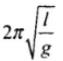where, l = length of the pendulum and
g = acceleration due to gravity on surface of the earth.
Thus,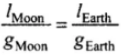or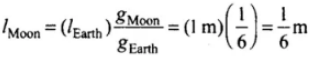Offer running on EduRev: Apply code STAYHOME200 to get INR 200 off on our premium plan EduRev Infinity!

291 docs

,

,

,

,

,

,

,

,

,

,

,

,

,

,

,

,

,

,

,

,

,

;